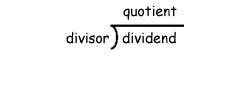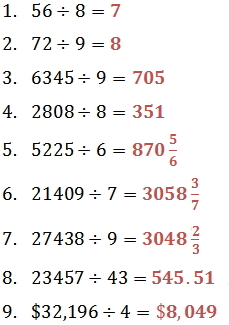Lesson - Divide Whole Numbers

Divide Whole Numbers

Division is used to separate objects into equal groups.

Dividend ÷ Divisor = QuotientKey Terms

• The dividend is the number that needs to be divided.
• The divisor shows the number of groups into which the dividend needs to be divided.
• The quotient is the answer, or result of the division. It shows the number of objects that will be in each group.

If you multiply the quotient by the divisor, its product will be the dividend. (Multiplication is an inverse operation of division.)

because 6 (quotient) x 4 (divisor) = 24 (dividend)

Example

Here are some important quotients and properties of division:

Important Quotients

Any number, except zero, divided by itself is 1.

Any number divided by 1 is that number.

Properties of Zero in Division

Zero divided by any other number is zero.

Division by zero is not allowed.

cannot be performed

There is no number whose product with 0 is 7.

Other Ways to Express Division

42 divided by 7 equals 6

42 / 7 = 6

When the dividend is a larger whole number, the digits in the quotient are found in steps. It is possible to check the answer using multiplication.

Example

Divide 844 by 5 and check.

Division with Remainders

Sometimes it is not possible to separate objects into a whole number of equal groups. In these cases, a few items will be left over, or remaining.

Practice

1.  Divide 56 by 8 and check.

2.  Divide 72 by 9 and check.

3.  Divide 6,345 by 9 and check.

4.  Divide 2,808 by 8 and check.

5.  Divide 5,225 by 6 and check.

6.  Divide 21,409 by 7 and check.

7.  Divide 27,438 by 9 and check.

8.  Find 23,457 divided by 43.  Round to the nearest hundred.

9.  Mrs. Garcia has \$32,196 she would like to will equally to 4 different charities.  About how much will each charity receive?